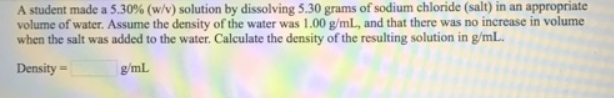# Problem: A student made a 5.30% (w/v) solution by dissolving 5.30 grams of sodium chloride (salt) in an appropriate volume of water. Assume the density of the water was 1.00 g/mL, and that there was no increase in volume when the salt was added to the water. Calculate the density of the resulting solution in g/mL.

🤓 Based on our data, we think this question is relevant for Professor Szymczyna's class at BU.

###### FREE Expert Solution###### Problem Details

A student made a 5.30% (w/v) solution by dissolving 5.30 grams of sodium chloride (salt) in an appropriate volume of water. Assume the density of the water was 1.00 g/mL, and that there was no increase in volume when the salt was added to the water. Calculate the density of the resulting solution in g/mL.